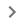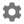# 设置 Gboard

## 下载 Gboard

• 在 Android 手机或平板电脑上安装 Gboard
• 在某些 Android 设备上，Gboard 已经是默认键盘。若要确保您的设备安装的是最新版本，请检查是否有更新

## 设置键盘选项

1. 在 Android 手机或平板电脑上，随意打开一个可以输入文字的应用，例如 Gmail 或 Keep。
2. 点按可以输入文字的区域。
3. 点按键盘左上角的“打开功能菜单”图标4. 依次点按“更多”图标“设置”图标5. 选择要开启的设置，例如滑行输入文字更正语音输入

1. 在 Android 手机或平板电脑上，随意打开一个可以输入文字的应用，例如 Gmail 或 Keep。
2. 点按可以输入文字的区域。
3. 点按键盘左上角的“打开功能菜单”图标4. 依次点按“更多”图标“设置”图标5. 点按搜索
6. 开启显示“搜索和其他功能”按钮

1. 在 Android 手机或平板电脑上，随意打开一个可以输入文字的应用，例如 Gmail 或 Keep。
2. 点按可以输入文字的区域。
3. 点按键盘左上角的“打开功能菜单”图标4. 依次点按“更多”图标“设置”图标语言
5. 选择所需语言。
6. 选择要使用的布局。
7. 点按完成

• 阿布哈兹语
• Aborlan 语
• 阿布朗语
• 亚齐语
• 阿乔利语
• 阿贾米语
• 阿达马瓦富尔富尔德语
• 阿迪格语
• 阿法尔语
• 南非语
• 阿希拉尼语
• 阿希拉尼语（音译）
• 阿贾格布语
• 阿坎语
• 阿库色语
• 阿拉斯语
• 阿尔巴尼亚语（盖格方言）
• 标准阿尔巴尼亚语
• 阿尔巴尼亚语（托斯克方言）
• 阿尔萨斯语
• 阿卢尔语
• 安汶马来语
• 安多藏语
• 阿姆哈拉语
• 阿美语
• 阿南格语
• 昂加语
• 阿拉伯语（阿尔及利亚）
• 阿拉伯语（巴林）
• 阿拉伯语（乍得）
• 阿拉伯语（埃及）
• 阿拉伯语（海湾）
• 阿拉伯语（伊拉克）
• 阿拉伯语（黎凡特）
• 阿拉伯语（利比亚）
• 阿拉伯语（毛里塔尼亚）
• 阿拉伯语（摩洛哥）
• 阿拉伯语（阿曼）
• 阿拉伯语（沙特阿拉伯）
• 阿拉伯语（苏丹）
• 阿拉伯语（突尼斯）
• 阿拉伯语（也门）
• 阿拉贡语
• 阿拉干语
• 亚美尼亚语
• 亚美尼亚语（音译）
• 阿萨姆语（阿萨姆文）
• 阿萨姆语（拉丁文）
• 阿萨姆语（音译）
• 亚述语（伊拉克）
• 亚述语（叙利亚）
• 阿斯图里亚斯语
• 奥地利-巴伐利亚语
• 阿瓦尔语
• 阿瓦德语
• 阿瓦德语（音译）
• 阿亚库乔克丘亚语
• 艾马拉语（玻利维亚）
• 艾马拉语（秘鲁）
• 阿塞拜疆语（阿塞拜疆）
• 阿塞拜疆语（伊朗）
• 阿塞拜疆语（俄罗斯）
• 巴哥里语
• 巴哥里语（音译）
• 巴格里语（印度）
• 巴格里语（印度、音译）
• 巴格里语（巴基斯坦）
• 巴格里语（巴基斯坦、音译）
• 巴哈马克里奥尔语
• 巴克提尔利语
• 巴厘语（巴厘字母）
• 巴厘语（拉丁文）
• 巴尔干嘎嘎乌兹土耳其语
• 巴尔干罗姆语（保加利亚）
• 巴尔干罗姆语（马其顿）
• 俾路支语
• 巴尔蒂语
• 巴尔蒂语（音译）
• 班巴拉语（拉丁文）
• 班巴拉语（西非书面字母）
• 巴姆穆语
• 班加拉语
• 邦盖语
• 邦加马来语
• 孟加拉语（孟加拉）
• 孟加拉语（印度、孟加拉文）
• 孟加拉语（印度、拉丁文）
• 孟加拉语（印度、音译）
• 班查语
• 巴乌雷语
• 巴萨语
• 巴什基尔语
• 巴斯克语
• 巴塔克曼特宁语（苏拉特巴塔克文）
• 巴塔克曼特宁语（拉丁文）
• 巴塔克西马隆贡语（苏拉特巴塔克文）
• 巴塔克西马隆贡语（拉丁文）
• 巴塔克托巴语
• 白俄罗斯语
• 奔巴语
• 本奇语（吉兹文）
• 本奇语（拉丁文）
• 贝罗姆语
• 巴达维语
• 比尔语（梵文）
• 比尔语（梵文、音译）
• 比尔语（古吉拉特文）
• 比尔语（古吉拉特文、音译）
• 博杰普尔语
• 博杰普尔语（音译）
• 比什奴普莱利亚语
• 比什奴普莱利亚语（音译）
• 比斯拉马语
• 博多语（孟加拉文）
• 博多语（孟加拉文、音译）
• 博多语（梵文）
• 博多语（梵文、音译）
• 博多语（拉丁文）
• 波斯尼亚语（西里尔文）
• 波斯尼亚语（拉丁文）
• 布依语
• 布拉灰语（阿拉伯文）
• 布拉灰语（拉丁文）
• 布拉灰语（音译）
• 布列塔尼语
• 布吉语（拉丁文）
• 布吉语（隆塔拉文）
• 布希德语
• 布库苏语
• 保加利亚语
• 布卢-贝内语
• 班德利语
• 班德利语（音译）
• 缅甸语
• 布里亚特语（蒙古）
• 布里亚特语（俄罗斯）
• 喀麦隆皮钦语
• 佛得角克里奥尔语
• 卡皮兹农语
• 加泰罗尼亚语
• 塞巴拉塞努福语
• 宿务语
• 中比科尔语
• 中部马库阿语
• 明古鲁语
• 查克马语
• 迦勒底新阿拉姆语
• 查莫罗语
• 查瓦卡诺语
• 车臣语
• 切罗基语（大写和小写）
• 切罗基语（仅大写）
• 恰蒂斯加尔语
• 恰蒂斯加尔语（音译）
• 钦博拉索克丘亚语
• 中文（粤语、繁体）
• 中文（普通话、简体）
• 中文（普通话、繁体）
• 吉大港语
• 吉大港语（音译）
• 乔克托语
• 乔克维语（安哥拉）
• 乔克维语（刚果民主共和国）
• Chopi 语
• 古教会斯拉夫语
• 楚瓦什语
• Chuwabu 语
• 古典叙利亚语
• 科普特语
• 康沃尔语
• 科西嘉语
• 克里语（拉丁文）
• 克里语（音节）
• 克里米亚鞑靼语（西里尔文）
• 克里米亚鞑靼语（拉丁文）
• 克罗地亚语
• 库斯科克丘亚语
• 库约农语
• 捷克语
• 达加拉语
• 达戈姆巴语
• 达基尼语
• 丹语
• 当梅语
• 丹麦语
• 达尔格瓦语
• 达里语
• 达萨语
• Dhatki 语
• 迪维希语
• 敦达语
• 敦达语（音译）
• 丁卡语
• 尤拉语（拉丁文）
• 尤拉语（西非书面字母）
• 多格来语（阿拉伯文）
• 多格来语（阿拉伯文、音译）
• 多格来语（梵文）
• 多格来语（梵文、音译）
• 多格来语（拉丁文）
• Dotyali 语
• 东干语
• Duri 语
• 荷兰语（比利时）
• 荷兰语（荷兰）
• 宗卡语
• 东法兰克语
• 东俾路支语
• 东占语（占文）
• 东占语（拉丁文）
• 闽东语
• 东奥罗莫语
• 东波克伦语
• 东达芒语
• 埃比拉语
• 伊多语
• 埃菲克语
• Elhomwe 语
• 艾米利亚语
• 英语（澳大利亚）
• 英语（加拿大）
• 英语（印度）
• 英语（肯尼亚）
• 英语（尼日利亚）
• 英语（菲律宾）
• 英语（南非）
• 英语（英国）
• 英语（美国）
• 厄尔兹亚语
• 世界语
• 爱沙尼亚语
• 埃维语
• 埃翁多语
• 埃斯特雷马杜拉语
• 芳语
• 芳蒂语
• Farefare 语
• 法罗语
• 斐济印地语（梵文）
• 斐济印地语（拉丁文）
• 斐济语
• 菲律宾语（贝贝因文）
• 菲律宾语（拉丁文）
• 芬兰语
• 丰族语
• 法语（非洲）
• 法语（比利时）
• 法语（加拿大）
• 法语（法国）
• 法语（瑞士）
• 弗留利语
• 富拉语（阿德拉姆文）
• 富拉语（拉丁文）
• 富拉语（尼日尔）
• 富拉语（尼日利亚）
• 加告兹语（西里尔文）
• 加告兹语（拉丁文）
• 加利西亚语
• Gamo 语
• 嘉华语
• 嘉华语（音译）
• 加罗语（孟加拉文）
• 加罗语（拉丁文）
• 加罗语（音译）
• 卡约语
• 吉兹语
• 盖德奥语
• 格鲁吉亚语（教士体）
• 格鲁吉亚语（骑士体）
• 德语（奥地利）
• 德语（比利时）
• 德语（德国）
• 德语（卢森堡）
• 德语（瑞士）
• 加纳皮钦语
• 戈马拉语
• 吉拉克语
• 吉利亚马语
• 哥达瓦里语
• 哥达瓦里语（音译）
• 戈戈语
• 冈德语（梵文）
• 冈德语（梵文、音译）
• 冈德语（泰卢固文）
• 冈德语（泰卢固文、音译）
• Gor 语
• 古拉尼语
• 哥伦打洛语
• 古尔芒切语
• 希腊语（塞浦路斯）
• 希腊语（希腊）
• 希腊语（希腊、音译）
• 瓜德罗普克里奥尔语
• 瓜拉尼语
• 圭亚那克里奥尔语
• 几内亚比绍克里奥尔语
• 古吉拉特语（古吉拉特文）
• 古吉拉特语（拉丁文）
• 古吉拉特语（音译）
• 古贾尔语（印度）
• 古贾尔语（印度、音译）
• 古贾尔语（巴基斯坦）
• 古贾尔语（巴基斯坦、音译）
• Gulay 语
• 古西语
• 圭亚那克里奥尔语
• 豪萨语
• 哈迪亚语（吉兹文）
• 哈迪亚语（拉丁文）
• 海地克里奥尔语
• 哈卡钦语
• 客家语
• 亥比语（梵文）
• 亥比语（梵文、音译）
• 亥比语（奥迪亚）
• 亥比语（奥迪亚、音译）
• 哈尼语
• 汉努诺奥语
• 哈劳提语
• 哈劳提语（音译）
• 哈里亚纳语
• 哈里亚纳语（音译）
• 豪萨语（加纳）
• 豪萨语（尼日利亚）
• 夏威夷语
• 夏威夷皮钦语
• 哈乌语
• 哈亚语
• 哈扎拉吉语
• 希伯来语
• Hehe 语
• 希利盖农语
• 山地马里语
• 印地语（梵文）
• 印地语（拉丁文/印度英语）
• 印地语（音译）
• 希里莫图语
• 苗语
• 白苗语
• 苗语
• 匈牙利语
• 洪斯吕克语
• 伊班语
• 伊比比奥语
• 冰岛语
• 伊加拉语
• 伊博语
• 伊洛果语
• 印度尼西亚语
• 印度科希斯坦语
• 英古什语
• 国际语
• 国际音标
• 因纽特语（拉丁文）
• 因纽特语（音节）
• 爱尔兰语
• 依善语
• 伊索科语
• 意大利语（意大利）
• 意大利语（瑞士）
• Itawit 语
• 勉语
• Izon 语
• 牙买加土语
• 占碑马来语
• 日语
• 爪哇语（爪哇字母）
• 爪哇语（班尤马文）
• 爪哇语（拉丁文）
• 景颇语
• 基切语
• 卡巴尔达语
• 卡比耶语
• 卡奇科里语
• 卡达山-杜顺语
• Kafa 语
• 格陵兰语
• 卡拉姆科希斯坦语
• 卡尔梅克卫拉特语
• 卡姆巴语
• 坎巴塔语（吉兹文）
• 坎巴塔语（拉丁文）
• 康格里语
• Kankanaey 语
• 卡纳达语（卡纳达文）
• 卡纳达语（拉丁文）
• 卡纳达语（音译）
• 卡瑙杰语
• 卡瑙杰语（音译）
• 卡努里语
• 邦板牙语
• 卡拉恰伊－巴尔卡尔语
• 卡拉卡尔帕克语（西里尔文）
• 卡拉卡尔帕克语（拉丁文）
• 基那来阿语
• 卡累利阿语
• 卡罗语
• 克什米尔语（阿拉伯文）
• 克什米尔语（阿拉伯文、音译）
• 克什米尔语（梵文）
• 克什米尔语（梵文、音译）
• 克什米尔语（拉丁文）
• 卡舒比语
• 哈萨克语（西里尔文）
• 吉打马来语
• 凯克其语
• 吉兰丹-北大年马来语
• 康巴藏语
• 卡西语
• 高棉语
• 高棉语（素辇府）
• 克木语
• 霍拉桑突厥语（阿拉伯文）
• 霍拉桑突厥语（拉丁文）
• 科瓦语
• Kiga 语
• 刚果语-吉土巴语
• 吉库尤语
• 金本杜语
• 卢旺达语
• 基普西吉语
• 基里巴斯
• 北扎扎其语
• 吉土巴语
• 科西斯坦辛那语
• 廓克博若克语（孟加拉文）
• 廓克博若克语（孟加拉文、音译）
• 廓克博若克语（拉丁文）
• 科隆语
• 科梅灵语
• 科米-彼尔米亚克语
• 科米-齐良语
• 刚果语
• 贡根语（梵文）
• 贡根语（梵文、音译）
• 贡根语（卡纳达文）
• 贡根语（卡纳达文、音译）
• 贡根语（拉丁文）
• 康孔巴语
• 孔索语
• 刚果语
• 韩语
• 科尔库语
• 科尔库语（音译）
• 科罗纳达尔-布拉安语
• 克里奥尔语
• Kuhmareyi 语
• 库伊语
• 库矛语
• 库矛语（音译）
• 库梅克语
• 库尔德语（伊朗、阿拉伯文）
• 库尔德语（伊拉克、阿拉伯文）
• 库尔德语（拉丁文）
• 库鲁克语（梵文）
• 库鲁克语（梵文、音译）
• 库鲁克语（马拉雅拉姆文）
• 库台马来语
• 喀奇语
• 库瓦亚马语
• 吉尔吉斯语
• 拉登语
• 拉迪诺语
• 拉迪诺语（以色列）
• 拉祜语
• 拉克语
• 拉基语
• 拉科塔语
• 拉玛语
• 兰巴迪语（梵文）
• 兰巴迪语（梵文、音译）
• 兰巴迪语（卡纳达文）
• 兰巴迪语（泰卢固文）
• 兰巴迪语（泰卢固文、音译）
• 楠榜阿皮语
• 兰戈语
• 老挝语
• 老挝语（音译）
• 拉特加莱语
• 拉丁语
• 拉脱维亚语
• 勒多-凯利语
• 伦杜语
• 雷布查语（雷布查文）
• 雷布查语（藏文）
• 列兹金语（阿塞拜疆）
• 列兹金语（俄罗斯）
• 利古里亚语
• 林布语（梵文）
• 林布语（林布文）
• 林堡语
• 林加拉语（安哥拉）
• 林加拉语（刚果民主共和国）
• 傈僳语
• 立陶宛语
• 利沃尼亚语
• 李维-卡累利阿语
• 逻辑语
• 伦巴第语
• 隆韦语
• 低地德语
• 下索布语
• 奇卢伯语
• 卢巴加丹加语
• 卢干达语
• 卢格巴拉语
• 卢奥语
• 卢森堡语
• 玛安晏语
• 马塞族语
• 马耶马耶语
• 马巴语（阿拉伯文）
• 马巴语（拉丁文）
• 马其顿语
• 马都拉语
• 摩揭陀语（印度）
• 摩揭陀语（印度、音译）
• 摩揭陀语（尼泊尔）
• 马京达瑙语
• 马哈拉施特拉邦孔卡尼语
• 马哈苏帕哈里语
• 迈蒂利语（梵文）
• 迈蒂利语（拉丁文）
• 迈蒂利语（音译）
• 望加锡马来语
• 望加锡语（拉丁文）
• 望加锡语（隆塔拉文）
• 马库阿-梅托语
• 马孔德语
• 马尔加什语
• 马来语（文莱、阿拉伯文）
• 马来语（文莱、拉丁文）
• 马来语（马来西亚、阿拉伯文）
• 马来语（马来西亚、拉丁文）
• 马来语（新加坡、拉丁文）
• 马拉雅拉姆语（拉丁文）
• 马拉雅拉姆语（马拉雅拉姆文）
• 马拉雅拉姆语（音译）
• 马耳他语
• 马尔维语
• 马尔维语（音译）
• 马马萨语
• 万鸦老马来语
• 曼迪安语
• 曼迪阿里语
• 曼迪阿里语（音译）
• 曼丁哥族语
• 芒加莱语
• 曼尼普尔语（孟加拉文）
• 曼尼普尔语（孟加拉文、音译）
• 曼尼普尔语（拉丁文）
• 曼尼普尔语（曼尼普尔）
• 曼尼普尔语（曼尼普尔、音译）
• 马恩岛语
• 毛利语
• 马拉瑙语
• 马拉地语（梵文）
• 马拉地语（拉丁文）
• 马拉地语（音译）
• 马尔巴语
• 马尔瓦里语（印度）
• 马尔瓦里语（印度、音译）
• 马尔瓦里语（巴基斯坦）
• 马尔瓦里语（巴基斯坦、音译）
• 马萨巴语
• 马萨语
• 马赞德兰语
• 草原马里语
• Mehri 语
• 马兰诺语
• 门德语
• 梅鲁语
• 马尔瓦里语
• 马尔瓦里语（音译）
• 梅瓦里语
• 梅瓦里语（音译）
• 米南佳保语
• 明格列尔语
• 米佐语
• Moba 语
• 莫克沙语
• 孟语
• 蒙古语
• 蒙古语（音译）
• 蒙贡多语
• 黑山语（西里尔文）
• 黑山语（拉丁文）
• 莫西语
• 穆纳语
• 蒙当语
• 蒙达里语（孟加拉文）
• 蒙达里语（梵文）
• 蒙达里语（拉丁文）
• 蒙达里语（奥里亚文）
• 芒语
• 穆西语
• 纳瓦特尔语
• 楠德语
• 南迪语
• 瑙鲁语
• 纳瓦霍语
• 恩道语
• 恩敦加语
• 那不勒斯语
• 涅涅茨语
• 尼泊尔语（印度、梵文）
• 尼泊尔语（印度、拉丁文）
• 尼泊尔语（印度、音译）
• 尼泊尔语（尼泊尔、梵文）
• 尼瓦尔语
• 恩加朱语
• Ngambay 语
• 格巴亚恩巴卡语
• 尼亚斯语
• 尼日利亚皮钦语
• 尼马地语
• 尼马地语（音译）
• 纽埃语
• 恩科勒语
• 特尔纳特马来语
• 北贝齐米萨拉卡-马拉加斯语
• 北格列博语
• 北欣德科语
• 北欣德科语（音译）
• 北卢尔语
• 闽北语
• 北恩德贝莱语
• 北萨米语
• 北索托语
• 北部泰语
• 挪威博克马尔语
• 挪威语（尼诺斯克文）
• 恩查姆语
• 侬语
• 诺苏语（拉丁文）
• 诺苏语（彝文）
• 努佩语
• 尼亚库萨语
• 尼亚姆韦齐语
• 尼扬贾语
• 尼亚图鲁语
• 尼奥罗语
• 奥克西唐语
• 奥里亚语（拉丁文）
• 奥里亚语（奥里亚文）
• 奥里亚语（音译）
• 奥杰布瓦语（拉丁文）
• 奥杰布瓦语（音节）
• 奥罗莫语
• 欧塞奇语
• 奥塞特语
• 巴奥克伦语
• 帕哈里-波特瓦里语
• 帕哈里-波特瓦里语（音译）
• 巴伦丁语（德国）
• 帕莫纳语
• 邦阿西楠语
• 帕皮阿门托语（阿鲁巴）
• 帕皮阿门托语（博内尔岛和库拉索岛）
• 巴布亚马来语
• 巴饶克佤语
• 帕尔卡里科里语
• 普什图语
• 普什图语（音译）
• 波斯语
• 波斯语（音译）
• 庇卡底语
• 皮埃蒙特语
• 平原马拉加斯语
• 波科特语
• 波兰语
• 本都希腊语
• 葡萄牙语（非洲）
• 葡萄牙语（安哥拉）
• 葡萄牙语（巴西）
• 葡萄牙语（澳门）
• 葡萄牙语（葡萄牙）
• 富拉语
• 旁遮普语（阿拉伯文）
• 旁遮普语（阿拉伯文、音译）
• 旁遮普语（果鲁穆奇文）
• 旁遮普语（果鲁穆奇文、音译）
• 旁遮普语（拉丁文）
• 卡什加语
• 克丘亚语
• 朗布尔语（孟加拉文）
• 朗布尔语（梵文）
• 朗布尔语（梵文、音译）
• 勒姜语
• 留尼汪克里奥尔语
• 林科纳达比考尔语
• 罗兴亚语
• 罗马尼亚语（摩尔多瓦）
• 罗马尼亚语（罗马尼亚）
• 罗曼什语
• 隆迪语
• 俄语（白俄罗斯）
• 俄语（吉尔吉斯斯坦）
• 俄语（俄罗斯）
• 卢森尼亚语
• 沙巴马来语
• 萨德里语
• 圣卢西亚克里奥尔法语
• 萨卡拉瓦-马拉加斯语
• 萨哈语
• 萨摩亚语
• 萨莫吉提亚语
• 桑戈语
• 梵语（梵文）
• 梵语（拉丁文）
• 梵语（音译）
• 桑塔利语（孟加拉文）
• 桑塔利语（孟加拉文、音译）
• 桑塔利语（梵文）
• 桑塔利语（拉丁文）
• 桑塔利语（欧甘文）
• 桑塔利语（欧甘文、音译）
• 萨拉语
• 西莱基语（信德阿拉伯文）
• 西莱基语（信德阿拉伯文、音译）
• 西莱基语（乌尔都文/阿拉伯文）
• 西莱基语（乌尔都文/阿拉伯文、音译）
• 撒丁尼亚语
• 萨萨克语（巴厘字母）
• 萨萨克语（拉丁文）
• 萨沙里语
• 索拉什特拉语
• 苏格兰语
• 苏格兰盖尔语
• 塞巴古拉格语
• 塞纳语
• 塞尔维亚语（西里尔字母）
• 塞尔维亚语（拉丁文）
• 塞雷尔语
• 塞索托语（莱索托）
• 塞索托语（南非）
• 塞舌尔克里奥尔语
• 斯高克伦语
• 掸语
• 沙卡瓦蒂语
• 沙卡瓦蒂语（音译）
• 夏尔巴语
• 希纳语
• 希纳语（音译）
• 修纳语
• 西伯利亚鞑靼语
• 西西里语
• 悉达摩语
• 西里西亚语
• 斯尔特语（吉兹文）
• 斯尔特语（拉丁文）
• 信德语（阿拉伯文）
• 信德语（阿拉伯文、音译）
• 信德语（梵文）
• 信德语（梵文、音译）
• 信德语（拉丁文）
• 僧伽罗语
• 僧伽罗语（音译）
• 斯洛伐克语
• 斯洛文尼亚语
• 索加语
• 索马里语
• Songe 语
• 索宁克语
• 南玻利维亚克丘亚语
• 东南部帕沙伊语
• 南俾路支语
• 南俾路支语（音译）
• 南贝齐米萨拉卡-马拉加斯语
• 南欣德科语
• 南欣德科语（音译）
• 南库尔德语
• 南卢尔语
• 闽南语 (POJ)
• 闽南语 (TL)
• 南恩德贝勒语
• 南奥罗莫语
• 南泰语
• 西南部帕沙伊语
• 西班牙语（阿根廷）
• 西班牙语（拉丁美洲）
• 西班牙语（墨西哥）
• 西班牙语（西班牙）
• 西班牙语（美国）
• 苏里南语
• 苏库马语
• 巽他语（阿拉伯文）
• 巽他语（拉丁文）
• 巽他语（巽他文）
• Supyire 语
• 苏尔古贾语
• 素拉杰普尔语
• 素拉杰普尔语（音译）
• 斯瓦希里语
• 斯威士语（南非）
• 斯威士语（斯威士兰）
• 瑞典语（芬兰）
• 瑞典语（瑞典）
• 瑞士德语
• 塞海蒂语（孟加拉文）
• 塞海蒂语（拉丁文）
• 塞海蒂语（塞洛提纳格瑞文）
• 塔巴萨兰语
• 塔希提语
• 黑傣语（拉丁文）
• 黑傣语（越南傣文）
• 傣仂语
• 傣那语
• 塔吉克语
• 塔里什语（阿塞拜疆）
• 塔里什语（伊朗）
• 塔里什语（俄罗斯）
• 塔马塞特语（拉丁文）
• 塔马齐格特语（提非纳文）
• 泰米尔语（印度、拉丁文）
• 泰米尔语（印度、泰米尔文）
• 泰米尔语（印度、音译）
• 泰米尔语（新加坡）
• 泰米尔语（斯里兰卡）
• 坦卓伊-马哈法利马拉加斯语
• 鞑靼语
• 塔特语
• 陶苏格语
• 岱语
• 泰卢固语（拉丁文）
• 泰卢固语（泰卢固文）
• 泰卢固语（音译）
• 提姆语
• 特索语
• 特特拉语
• 德顿语
• 泰语
• 藏语
• 蒂格尼亚语（厄立特里亚）
• 蒂格尼亚语（埃塞俄比亚）
• 提夫语
• 巴布亚皮钦语
• 托克劳语
• 汤加语
• 土柔语
• 托拉查语
• 托尔瓦里语
• 特立尼达克里奥尔英语
• 宗加语
• Tswa 语
• 茨瓦纳语（博茨瓦纳）
• 茨瓦纳语（南非）
• 图鲁语
• 图鲁语（音译）
• 图姆布卡语
• 图尔卡纳语
• 土耳其语（塞浦路斯）
• 土耳其语（土耳其）
• 土库曼语
• 图瓦卢语
• 图瓦语
• 乌德穆尔特语
• 乌克兰语
• 姆班杜语
• 上撒克逊语
• 上索布语
• 乌尔都语（印度）
• 乌尔都语（印度、拉丁文）
• 乌尔都语（印度、音译）
• 乌尔都语（巴基斯坦）
• 乌鲁姆语
• 维吾尔语
• 乌兹别克语（西里尔文）
• 乌兹别克语（拉丁文）
• 瓦伊语
• 巴伦西亚语
• 瓦哈第语
• 瓦哈第语（音译）
• 瓦萨维语（梵文）
• 瓦萨维语（古吉拉特文）
• 古诺尔斯语
• 文达语
• 威尼斯语
• 维普森语
• 越南语
• 弗拉赫罗姆语（阿尔巴尼亚）
• 夫拉克斯罗姆语（罗马尼亚）
• 佛罗语
• 瓦格迪语
• 瓦格迪语（音译）
• 瓦隆语
• 瓦济里语
• 瓦瑞语
• 瓦尤语
• 威尔士语
• 西佛兰德语
• 西俾路支语
• 西占语（占文）
• 西占语（拉丁文）
• 西弗里西亚语
• 西克耶语（克耶黎文）
• 瓦拉莫语
• 沃洛夫语
• 哈松格克桑格语
• 科萨语
• 湘西苗语
• 雅卡语
• 瑶语
• 意第绪语（以色列）
• 意第绪语（美国）
• 意第绪语（美国、音译）
• 邕北壮语
• 约鲁巴语
• 右江壮语
• 尤卡坦玛雅语
• 赞德语
• 哲尔马语
• 扎扎语
• 西兰语
• 壮语
• 祖鲁语